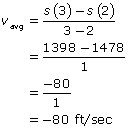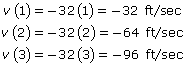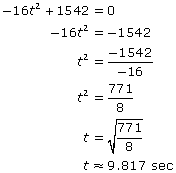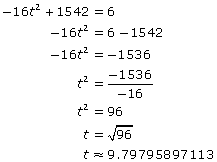# Problem 3: Position and Velocity

2011-2012 An object is dropped from the second-highest floor of the Sears Tower, 1542 feet off of the ground. (The top floor was unavailable, occupied by crews taping for the new ABC special “Behind the Final Behind the Rose Final Special, the Most Dramatic Behind the Special Behind the Rose Ever.”) (a) Construct the position … Continue reading "Problem 3: Position and Velocity"##2011-2012

An object is dropped from the second-highest floor of the Sears Tower, 1542 feet off of the ground. (The top floor was unavailable, occupied by crews taping for the new ABC special “Behind the Final Behind the Rose Final Special, the Most Dramatic Behind the Special Behind the Rose Ever.”)

(a) Construct the position and velocity equations for the object in terms of t, where t represents the number of seconds that have elapsed since the object was released.

(b) Calculate the average velocity of the object over the interval t = 2 and t = 3 seconds.

(c) Compute the velocity of the object 1, 2, and 3 seconds after it is released.

(d) How many seconds does it take the object to hit the ground? Report your answer accurate to the thousandths place.

(e) If the object were to hit a six-foot-tall man squarely on the top of the head as he (unluckily) passed beneath, how fast would the object be moving at the moment of impact? Report your answer accurate to the thousandths place.

Extra Credit: If the falling object killed the six-foot-tall man, is he actually luckier for not having to endure the Bachelor special taping on the top floor? (Spoiler alert: Yes.)

### Solution:

(a) The position function for a projectile is s(t) = –16t2 + v0t + h0, where v0  represents the initial velocity of the object (in this case 0) and h0 represents the initial height of the object (in this case 1,542 feet). Note that this position equation represents the height in feet of the object t seconds after it is released. Thus, the position equation is s(t) = –16t2 + 1,542. The vecocity equation v(t) is the derivative of the position equation: v(t) = –32t.

## How to Find Average Velocity

(b) Average velocity is the slope of the secant line, rather than the slope of the tangent line. Finding average velocity is easy. Plug t = 2 and t = 3 into the position equation to calculate the height of the object at the boundaries of the indicated interval to generate two ordered pair: (2, 1478) and (3, 1398). Apply the slope formula from basic algebra to calculate the slope of the line passing through those points.(c) Substitute t = 1, 2, and 3 into v(t).(d) The object hits the ground when its position is s(t) = 0. Set the position equation equal to zero and solve for t.(e) The problem asks you to calculate the velocity of the object when it is exactly six feet off of the ground, when s(t) = 6. Apply the same technique you completed in part (d), but instead of calculating the time t when the object’s position is 0, calculate the time t when its position is 6.Now calculate the velocity of the object at that time: v(9.79795897113) = –32(9.79795897113) = –313.535 ft/sec.

The video may take a few seconds to load.Having trouble Viewing Video content? Some browsers do not support this version – Try a different browser.